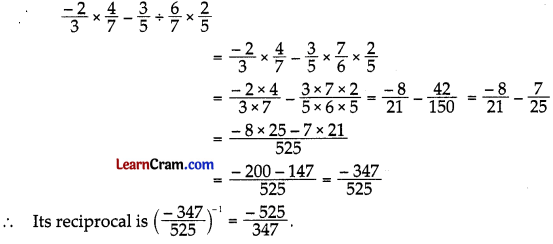# DAV Class 7 Maths Chapter 2 Brain Teasers Solutions

The DAV Class 7 Maths Book Solutions Pdf and DAV Class 7 Maths Chapter 2 Brain Teasers Solutions of Operations on Rational Numbers offer comprehensive answers to textbook questions.

## DAV Class 7 Maths Ch 2 Brain Teasers Solutions

Question 1.
A. Tick (✓) the correct option:
(i) The additive inverse of $$\frac{-3}{4}$$ is
(a) $$\frac{-3}{4}$$
(b) $$\frac{-4}{3}$$
(c) $$\frac{4}{3}$$
(d) $$\frac{3}{4}$$
(d) $$\frac{3}{4}$$

If $$\frac{-3}{4}$$ is a rational number, then -($$\frac{-3}{4}$$) i.e, $$\frac{3}{4}$$ is the additive inverse of $$\frac{-3}{4}$$ such that $$\frac{-3}{4}+\left[-\left(\frac{-3}{4}\right)\right]=\frac{-3}{4}+\left(\frac{-3}{4}\right)$$ = 0

(ii) If x, y and z are rational numbers, then the property (x + y) + z = x + (y + z) is known as
(a) communicative property
(b) associative property
(c) distributive property
(d) closure property
(b) associative property

Sum of three rational numbers remains same even of after changing the grouping of the addends, i.e., if x, y and z are three rational numbers then (x + y) + z = x + (y + z) This is known as associative law of addition.
Hence, (b) is the correct answer.

(iii) $$\frac{7}{12} \div\left(\frac{-7}{12}\right)$$ is
(a) 1
(b) 7
(c) -1
(d) does not exist
(c) -1

$$\frac{7}{12} \div\left(\frac{-7}{12}\right)$$
= $$\frac{7}{12} \times\left(\frac{-12}{7}\right)$$
= -1

Hence, (c) is the correct answer.

(iv) Identity element for subtraction of rational numbers is
(a) 1
(b) 0
(c) -1
(d) -7
(d) -7

For all rational numbers x, we have
x – 0 = x
But, 0 – x = -x
Therefore, identity element for subtraction of rational numbers does not exist.
Hence, (d) is the correct answer.

(v) The multiplicative inverse of 6$$\frac{1}{3}$$ is
(a) $$\frac{-19}{3}$$
(b) $$\frac{-3}{19}$$
(c) $$\frac{3}{19}$$
(d) $$\frac{19}{3}$$
(c) $$\frac{3}{19}$$

6$$\frac{1}{3}$$ = $$\frac{19}{3}$$
So, by simply interchanging the numerator and denominator, we can find the multiplicative inverse.
Therefore, multiplicative inverse of 6$$\frac{1}{3}$$ i.e., $$\frac{19}{3}$$ is $$\frac{3}{19}$$.
Hence, (c) is the correct answer.(i) Write all rational numbers whose absolute value is $$\frac{5}{9}$$.
The absolute value of a rational number written in the following ways.
Absolute value of $$\frac{5}{9}$$ is $$\left|\frac{5}{9}\right|=\frac{5}{9}$$
Absolute value of $$\frac{-5}{9}$$ is $$\left|\frac{-5}{9}\right|=\frac{5}{9}$$
Therefore, $$\frac{5}{9}$$ and $$\frac{-5}{9}$$ are the rational numbers whose absolute value is $$\frac{5}{9}$$

(ii) Find the reciprocal of $$\frac{4}{5} \times\left(\frac{3}{-8}\right)$$
$$\frac{4}{5} \times\left(\frac{3}{-8}\right)$$
= $$\frac{4 \times 3}{5 \times(-8)}$$ [Divide 4 and (-8) by 4 to get rational number in standard form].
= $$\frac{1 \times 3}{5 \times(-2)}=\frac{3}{(-10)}=\frac{-3}{10}$$
Now, by simply interchanging the numerator and denominator, we can find reciprocal.
∴ Reciprocal of $$\frac{-3}{10}$$ is $$\frac{10}{-3}$$,i.e., $$\frac{-10}{3}$$.

(iii) What should be added to $$\frac{-5}{11}$$ to get $$\frac{26}{33}$$
Here, the sum of the two numbers is $$\frac{26}{33}$$ and one of them is $$\frac{-5}{11}$$.
Required number = $$\frac{26}{33}-\left(\frac{-5}{11}\right)$$
= $$\frac{26}{33}+\frac{5}{11}=\frac{26+15}{33}=\frac{41}{33}$$

Hence, the required number is $$\frac{41}{33}$$.

(iv) Subtract 6$$\frac{2}{3}$$ from the sum of $$\frac{-3}{7}$$ and 2.
Sum of $$\frac{-3}{7}$$ and 2 = $$\frac{-3}{7}$$ + 2
= $$\frac{-3}{7}+\frac{2}{1}=\frac{-3+14}{7}=\frac{11}{7}$$

Now, we have to subtract 6$$\frac{2}{3}$$ i.e., $$\frac{20}{3}$$ from $$\frac{11}{7}$$.
⇒ $$\frac{11}{7}-\frac{20}{3}=\frac{33-140}{21}=\frac{-107}{21}$$

(v) Find the value of 1 + $$\frac{1}{1+\frac{1}{6}}$$
1 + $$\frac{1}{1+\frac{1}{6}}$$
= 1 + $$\frac{1}{\left(\frac{1}{1}+\frac{1}{6}\right)}$$
= 1 + $$\frac{1}{\left(\frac{6+1}{6}\right)}$$
= 1 + $$\frac{1}{\left(\frac{7}{6}\right)}$$
= 1 + (1 × $$\frac{6}{7}$$)
= 1 + $$\frac{6}{7}$$
= $$\frac{7+6}{7}$$
= $$\frac{13}{7}$$

Question 2. State whether the following statements are true or false. If false, justify your answer with an example.
(i) If |x| = 0, then x has no reciprocal.
True

(ii) If x < y then |x| < |y|
False.

Justification:
Take x = – 4 and y = – 3
– 4 < – 3 but |-4| > |-3| = 4 > 3
So it is false.

(iii) If x-1 < y-1
False.
Justification:
Take x = 3 and y = 4
Here 3 < 4 and $$\frac{1}{3}>\frac{1}{4}$$

(iv) The negative of a negative rational number is a positive rational number.
True

(v) Product of two rational numbers can never be an integer.
False

Justification:
Take x = $$\frac{2}{3}$$ and y = $$\frac{-3}{2}$$
∴ xy = $$\frac{2}{3} \times \frac{-3}{2}$$ = -1 integer

(vi) Product of two integers is never a fraction.
True

(vii) If x and y are two rational numbers such that x > y then x – y is always a positive rational number.
TrueQuestion 3.
For x = $$\frac{3}{4}$$ and y = $$\frac{-9}{8}$$, insert a rational number between:
(i) (x + y)-1 and x-1 + y -1
(x + y) -1 = $$\left(\frac{3}{4}+\frac{-9}{8}\right)^{-1}=\left(\frac{3}{4}-\frac{9}{8}\right)^{-1}$$
= $$\left(\frac{3 \times 2-9 \times 1}{8}\right)^{-1}$$
= $$\left(\frac{6-9}{8}\right)^{-1}=\left(\frac{-3}{8}\right)^{-1}$$
= $$\frac{-8}{3}$$

x-1 + y-1 = 
= $$\left(\frac{3}{4}\right)^{-1}+\left(\frac{-9}{8}\right)^{-1}$$
= $$\frac{4}{3}-\frac{8}{9}=\left(\frac{4 \times 3-8 \times 1}{9}\right)$$
= $$\frac{12-8}{9}=\frac{4}{9}$$

Rational number between  and 
= $$\frac{1}{2}\left(\frac{-8}{3}+\frac{4}{9}\right)$$
= $$\frac{1}{2}\left(\frac{-8 \times 3+4 \times 1}{9}\right)$$
= $$\frac{1}{2}\left(\frac{-24+4}{9}\right)$$
= $$\frac{1}{2} \times \frac{-2}{9}=\frac{-10}{9}$$

Hence the required rational number = $$\frac{-10}{9}$$

(ii) (x – y)-1 and x-1 – y-1
(x – y)-1 = $$\left(\frac{3}{4}-\frac{-9}{8}\right)^{-1}$$
= $$\left(\frac{3}{4}+\frac{9}{8}\right)^{-1}\left(\frac{3 \times 2+9 \times 1}{8}\right)^{-1}$$
= $$\left(\frac{6+9}{8}\right)^{-1}=\left(\frac{15}{18}\right)^{-1}$$
= $$\frac{8}{15}$$

x-1 – y-1 = $$\left(\frac{3}{4}\right)^{-1}-\left(\frac{-9}{8}\right)^{-1}$$
= $$\frac{4}{3}-\left(\frac{-8}{9}\right)$$
= $$\left(\frac{4}{3}+\frac{8}{9}\right)$$
= $$\left(\frac{4 \times 3+8 \times 1}{9}\right)$$
= $$\frac{12+8}{9}=\frac{20}{9}$$

Rational number between $$\frac{8}{15}$$ and $$\frac{20}{9}$$
= $$\frac{1}{2}\left(\frac{8}{15}+\frac{20}{9}\right)$$
= $$\frac{1}{2}\left(\frac{3 \times 8+20 \times 5}{45}\right)$$
= $$\frac{1}{2}\left(\frac{24+100}{45}\right)$$
= $$\frac{1}{2} \times \frac{124}{45}=\frac{62}{45}$$

Hence the required rational number is $$\frac{62}{45}$$

Question 4.
Verify that: (x ÷ y)-1 = x-1 ÷ y-1 by taking x = $$\frac{-5}{11}$$, y = $$\frac{7}{3}$$.
L.H.S = (x ÷ y)-1 = $$\left(\frac{-5}{11} \div \frac{7}{3}\right)^{-1}$$
= $$\left(\frac{-5}{11} \times \frac{3}{7}\right)^{-1}$$
= $$\left(\frac{-5 \times 3}{11 \times 7}\right)^{-1}$$
= $$\left(\frac{-15}{77}\right)^{-1}$$
= $$\frac{77}{-15}$$

R.H.S = x-1 ÷ y-1 = $$\left(\frac{-5}{11}\right)^{-1} \div\left(\frac{7}{3}\right)^{-1}$$
= $$\frac{11}{-5} \div \frac{3}{7}$$
= $$\frac{11}{-5} \times \frac{7}{3}$$
= $$\frac{11 \times 7}{-5 \times 3}$$
= $$\frac{77}{-15}$$

L.H.S = R.H.S
Hence Verified.

Question 5.
Verify that |x + y| ≤ |x| + |y| by taking x = $$\frac{2}{3}$$, y = $$\frac{-3}{5}$$
|x + y| = $$\left|\frac{2}{3}+\left(\frac{-3}{5}\right)\right|$$
= $$\left|\frac{2}{3}-\frac{3}{5}\right|$$
= $$\left|\frac{10-9}{15}\right|$$
= $$\left|\frac{1}{15}\right|$$
= $$\frac{1}{15}$$

Now, |x| + |y| = $$\left|\frac{2}{3}\right|+\left|\frac{-3}{5}\right|$$
= $$\frac{2}{3}+\frac{3}{5}$$
= $$\frac{10+9}{15}$$
= $$\frac{19}{15}$$

As $$\frac{1}{15}<\frac{19}{15}$$, so |x + y| ≤ |x| + |y|
Hence, verified.

Question 6.
Find the reciprocals of:
(i) $$\frac{2}{-5} \times \frac{3}{-7}$$
Reciprocal of
$$\frac{2}{-5} \times \frac{3}{-7}$$
= $$\left(\frac{2}{-5} \times \frac{3}{-7}\right)^{-1}$$
= $$\left(\frac{2 \times 3}{-5 \times-7}\right)^{-1}$$
= $$\left(\frac{6}{35}\right)^{-1}=\frac{35}{6}$$

(ii) $$\frac{-4}{3} \times \frac{-5}{-8}$$
Reciprocal of
$$\left(\frac{6}{35}\right)^{-1}=\frac{35}{6}$$
= $$\left(\frac{-4 x-5}{3 \times-8}\right)^{-1}$$
= $$\left(\frac{20}{-24}\right)^{-1}$$
= $$\frac{-24}{20}=\frac{-6}{5}$$ (Standard form)

Question 6.
Simplify:
(i) $$\left|\frac{5}{7}-\frac{2}{3}\right|+\left|\frac{3}{14}-\frac{5}{7}\right|$$(ii) $$\left(\frac{5}{11}\right)^{-1}-\frac{13}{5}+\frac{3}{15}$$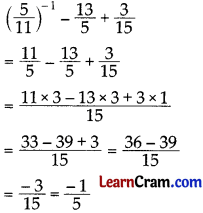(iii) $$\frac{9}{5} \times \frac{-2}{27}+\frac{7}{30}$$(iv) $$\frac{-7}{15} \div\left(\frac{50}{3}\right)^{-1}$$
$$\frac{-7}{15} \div\left(\frac{50}{3}\right)^{-1}$$
= $$\frac{-7}{15} \div\left(\frac{3}{50}\right)$$
= $$\frac{-7}{15} \times \frac{50}{3}$$
= $$\frac{-70}{9}$$Question 7.
Divide:
(i) The sum of $$\frac{5}{21}$$ and $$\frac{4}{7}$$ by their difference.
Sum = $$\frac{5}{21}+\frac{4}{7}$$
= $$\frac{5 \times 1+4 \times 3}{21}$$
= $$\frac{5+12}{21}$$
= $$\frac{17}{21}$$

Difference = $$\frac{5}{21}-\frac{4}{7}$$
= $$\frac{5 \times 1-4 \times 3}{21}$$
= $$\frac{5-12}{21}=\frac{-7}{21}$$

Now $$\frac{17}{21} \div \frac{-7}{21}$$
= $$\frac{17}{21} \times \frac{21}{-7}$$
= $$\frac{-17}{7}$$

(ii) The difference of $$\frac{12}{5}, \frac{-16}{20}$$ by their product.
Difference of $$\frac{12}{5}$$ and $$\frac{-16}{20}$$
= $$\frac{12}{5}-\left(\frac{-16}{20}\right)$$
= $$\frac{12}{5}+\frac{16}{20}$$
= $$\frac{12 \times 4+16 \times 1}{20}$$
= $$\frac{48+16}{20}$$
= $$\frac{64}{20}$$
= $$\frac{16}{5}$$

Product of $$\frac{12}{5}$$ and $$\frac{-16}{20}$$
= $$\frac{12}{5} \times \frac{-16}{20}$$
= $$\frac{-48}{25}$$

∴ $$\frac{16}{5} \div \frac{-48}{25}$$
= $$\frac{16}{5} \times \frac{-25}{48}$$
= $$\frac{-5}{3}$$

Question 8.
Find the reciprocal of $$\frac{-2}{3} \times \frac{5}{7}+\frac{2}{9} \div \frac{1}{3} \times \frac{6}{7}$$
$$\frac{-2}{3} \times \frac{5}{7}+\frac{2}{9} \div \frac{1}{3} \times \frac{6}{7}$$
= $$\frac{-2}{3} \times \frac{5}{7}+\frac{2}{9} \div \frac{1}{3} \times \frac{6}{7}$$
= $$\frac{-10}{21}+\frac{4}{7}$$
= $$\frac{-10+12}{21}$$
= $$\frac{2}{21}$$

∴ Its reciprocal = $$\left(\frac{2}{21}\right)^{-1}=\frac{21}{2}$$

## DAV Class 7 Maths Chapter 2 HOTS

Question 1.
A drum of kerosene oil is $$\frac{3}{4}$$ full. When 15 litres of oil is drawn from it, it is $$\frac{7}{12}$$ full the total capacity of the drum.
Let the total capacity of the drum be x litres.
According to the question, we have
$$\frac{3}{4}$$x – 15 = $$\frac{7}{12}$$x
⇒ $$\frac{3}{4}$$x – $$\frac{7}{12}$$x = 15
⇒ $$\frac{9 x-7 x}{12}$$ = 15
⇒ $$\frac{2x}{12}$$ = 15
⇒ x = $$\frac{12 \times 15}{2}$$
⇒ x = 6 × 15 = 90
Therefore, the total capacity of the drum is 90 litres.

Question 2.
Find the product of:
(1 – $$\frac{1}{2}$$)(1 – $$\frac{1}{3}$$)(1 – $$\frac{1}{4}$$) ……… (1 – $$\frac{1}{10}$$)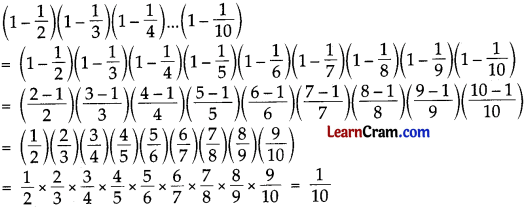## DAV Class 7 Maths Chapter 2 Enrichment Questions

Question 1.
Complete the following magic square of multiplication.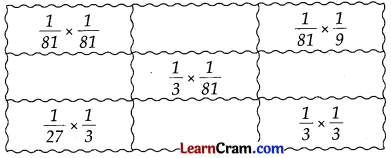Question 1.
Find equivalent forms of rational numbers having a common denominator in the given group of rational numbers. $$\frac{5}{8}, \frac{6}{9}, \frac{5}{36}, \frac{13}{30}$$
Solution:
L.C.M. of 8, 9, 36, 30 = 360
= $$\frac{5 \times 45}{8 \times 45}, \frac{6 \times 40}{9 \times 40}, \frac{5 \times 10}{36 \times 10}, \frac{13 \times 12}{30 \times 12}$$
= $$\frac{225}{360}, \frac{240}{360}, \frac{50}{360}, \frac{156}{360}$$

Question 2.
Verify commutative property for x = $$\frac{-2}{7}$$ and y = $$\frac{3}{5}$$.
Commutative property for rational numbers is
x + y = y + x
L.H.S. = x + y = $$\frac{-2}{7}+\frac{3}{5}$$
= $$\frac{-2 \times 5+3 \times 7}{35}$$
= $$\frac{-10+21}{35}$$
= $$\frac{11}{35}$$

R.H.S = y + x = $$\frac{3}{5}+\left(\frac{-2}{7}\right)$$
= $$\frac{3}{5}-\frac{2}{7}$$
= $$\frac{3 \times 7-2 \times 5}{35}$$
= $$\frac{21-10}{35}$$
= $$\frac{11}{35}$$

L.H.S. = R.H.S.
Hence verified.

Question 3.
Verify associative property for rational number if x = $$\frac{3}{8}$$, y = $$\frac{5}{6}$$ and z = $$\frac{-2}{3}$$.
Associative property for rational numbers is
x + (y + z) = (x + y) + z

L.H.S = x + (y + z) = $$\frac{3}{8}+\left(\frac{5}{6}+\frac{-2}{3}\right)$$
= $$\frac{3}{8}+\left(\frac{5}{6}-\frac{2}{3}\right)$$
= $$\frac{3}{8}+\left(\frac{5 \times 1-2 \times 2}{6}\right)$$
= $$\frac{3}{8}+\left(\frac{5-4}{6}\right)$$
= $$\frac{3}{8}+\frac{1}{6}$$
= $$\frac{3 \times 3+1 \times 4}{24}$$
= $$\frac{9+4}{24}$$
= $$\frac{13}{24}$$

R.H.S = (x + y) + z = $$\left(\frac{3}{8}+\frac{5}{6}\right)+\left(\frac{-2}{3}\right)$$
= $$\left(\frac{3 \times 3+5 \times 4}{24}\right)-\frac{2}{3}$$
= $$\left(\frac{9+20}{24}\right)-\frac{2}{3}$$
= $$\frac{29}{24}-\frac{2}{3}$$
= $$\frac{29 \times 1-2 \times 8}{24}$$
= $$\frac{29-16}{24}$$
= $$\frac{13}{24}$$

L.H.S. = R.H.S. Hence verified.Question 4.
Find the value of x × (y + z) and x × y + x × z. State the property held there in given expressions, if x = $$\frac{5}{7}$$, y = $$\frac{3}{-4}$$ and z = $$\frac{2}{5}$$
x × (y + z) = $$\frac{5}{7} \times\left(\frac{-3}{4}+\frac{2}{5}\right)$$
= $$\frac{5}{7} \times\left(\frac{-3 \times 5+2 \times 4}{20}\right)$$
= $$\frac{5}{7} \times\left(\frac{-15+8}{20}\right)$$
= $$\frac{5}{7} \times \frac{-7}{20}=-\frac{1}{4}$$

x × y + x × z = $$\frac{5}{7} \times \frac{-3}{4}+\frac{5}{7} \times \frac{2}{5}$$
= $$\frac{5 \times-3}{7 \times 4}+\frac{5 \times 2}{7 \times 5}$$
= $$\frac{-15}{28}+\frac{10}{35}$$
= $$\frac{-15 \times 5+4 \times 10}{140}$$
= $$\frac{-75+40}{140}=\frac{-35}{140}$$
= $$\frac{-1}{4}$$

Here x × (y + z) = x × y + x × z = $$\frac{-1}{4}$$
∴ It is distribution of multiplication over addition property for rational numbers.

Question 5.
Find the value of the following $$\frac{3}{5} \times \frac{3}{4} \div \frac{9}{8}+\frac{3}{5}-\frac{4}{11}$$ × 0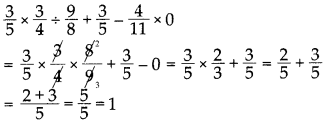Hence the required value is 1.

Question 6.
State whether x – y = y – x for x = $$\frac{-3}{7}$$ and y = $$\frac{2}{5}$$.
L.H.S = x – y = $$\frac{-3}{7}-\frac{2}{5}$$
= $$\frac{-3 \times 5-2 \times 7}{35}$$
= $$\frac{-15-14}{35}$$
= $$\frac{-29}{35}$$

R.H.S = y – x = $$\frac{2}{5}-\left(\frac{-3}{7}\right)$$
= $$\frac{2}{5}+\frac{3}{7}$$
= $$\frac{2 \times 7+3 \times 5}{35}$$
= $$\frac{14+15}{35}$$
= $$\frac{29}{35}$$

L.H.S = R.H.S
So they are not equal.

Question 7.
The sum of two rational numbers is – 3. If one of them is $$\frac{-2}{7}$$, find the other number.
Solution:
Sum of the two rational numbers = – 3
other number = $$\frac{-2}{7}$$
= -3 + ($$\frac{-2}{7}$$)
= -3 + $$\frac{2}{7}$$
= $$\frac{-3}{1}+\frac{2}{7}$$
= $$\frac{-3 \times 7+2 \times 1}{7}$$
= $$\frac{-21+2}{7}$$
= $$\frac{-19}{7}$$

Question 8.
For x = $$\frac{5}{6}$$, y = $$\frac{-3}{4}$$ and z = $$\frac{1}{2}$$ verify x × (y – z) = x × y – x × z
L.H.S
x × (y – z) = $$\frac{5}{6} \times\left(\frac{-3}{4}-\frac{1}{2}\right)$$
= $$\frac{5}{6} \times\left(\frac{-3 \times 1-1 \times 2}{4}\right)$$
= $$\frac{5}{6} \times\left(\frac{-3-2}{4}\right)$$
= $$\frac{5}{6} \times \frac{-5}{4}=\frac{5 \times-5}{6 \times 4}$$
= $$\frac{-25}{24}$$

R.H.S
x × y – x × z = $$\frac{5}{6} \times \frac{-3}{4}-\frac{5}{6} \times \frac{1}{2}$$
= $$\frac{5 \times-3}{6 \times 4}-\frac{5 \times 1}{6 \times 2}$$
= $$\frac{-15}{24}-\frac{5}{12}$$
= $$\frac{-15-5 \times 2}{24}$$
= $$\frac{-15-10}{24}$$
= $$\frac{-25}{24}$$

L.H.S = R.H.S
Hence verified.Question 9.
Verify that (x + y)-1 ≠ x-1 + y-1 if x = $$\frac{2}{5}$$, y = $$\frac{-1}{3}$$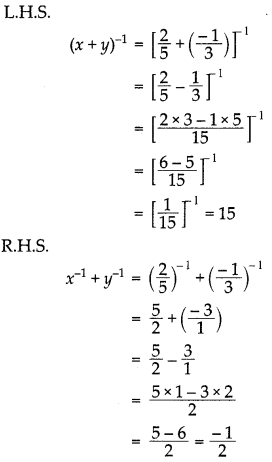L.H.S ≠ R.H.S
Hence verified

Question 10.
If x = $$\frac{2}{5}$$, y = $$\frac{-1}{3}$$ verify that (x – y)-1 ≠ x-1 – y-1L.H.S ≠ R.H.S
Hence verified

Question 11.
Verify that (x × y)-1 = x-1 × y-1 for x = $$\frac{2}{11}$$ and y = $$\frac{-3}{7}$$
L.H.S = (x × y)-1
= $$\left(\frac{2}{11} \times \frac{-3}{7}\right)^{-1}$$
= $$\left(\frac{2 \times-3}{11 \times 7}\right)^{-1}=\left(\frac{-6}{77}\right)^{-1}$$
= $$\frac{77}{-6}=\frac{-77}{6}$$

R.H.S = x-1 × y-1
= $$\left(\frac{2}{11}\right)^{-1} \times\left(\frac{-3}{7}\right)^{-1}$$
= $$\frac{11}{2} \times \frac{-7}{3}$$
= $$\frac{11 \times-7}{2 \times 3}$$
= $$\frac{-77}{6}$$

Question 12.
For x = $$\frac{4}{5}$$, y = $$\frac{2}{9}$$ and z = $$\frac{1}{3}$$ verify that (x + y) ÷ z = x ÷ z + y ÷ z.Question 13.
State whether (x ÷ y) ÷ z = x ÷ (y ÷ z) for x = $$\frac{2}{3}$$, y = $$\frac{1}{4}$$ and z = $$\frac{1}{2}$$.
L.H.S = (x ÷ y) ÷ z
= $$\left(\frac{2}{3} \div \frac{1}{4}\right) \div \frac{1}{2}$$
= $$\left(\frac{2}{3} \times \frac{4}{1}\right) \div \frac{1}{2}$$
= $$\left(\frac{2 \times 4}{3 \times 1}\right) \div \frac{1}{2}$$
= $$\frac{8}{3} \div \frac{1}{2}=\frac{8}{3} \times \frac{2}{1}$$
= $$\frac{16}{3}$$

R.H.S = x ÷ (y ÷ z)
= $$\frac{2}{3} \div\left(\frac{1}{4} \div \frac{1}{2}\right)$$
= $$\frac{2}{3} \div\left(\frac{1}{4} \times \frac{2}{1}\right)$$
= $$\frac{2}{3} \div\left(\frac{1 \times 2}{4 \times 1}\right)$$
= $$\frac{2}{3} \div \frac{2}{4}=\frac{2}{3} \times \frac{4}{2}$$
= $$\frac{4}{3}$$

L.H.S ≠ R.H.S
So, (x ÷ y) ÷ z ≠ x ÷ (y ÷ z)

Question 14.
Find three rational number between $$\frac{-2}{3}$$ and $$\frac{1}{3}$$
First rational number between $$\frac{-2}{3}$$ and $$\frac{1}{3}$$
= $$\frac{1}{2}\left(\frac{-2}{3}+\frac{1}{3}\right)$$
= $$\frac{1}{2}\left(\frac{-2+1}{3}\right)$$
= $$\frac{1}{2} \times \frac{-1}{3}=\frac{-1}{6}$$

Second rational number between $$\frac{-2}{3}$$ and $$\frac{-1}{6}$$
= $$\frac{1}{2}\left(\frac{-2}{3}-\frac{1}{6}\right)$$
= $$\frac{1}{2}\left(\frac{-2 \times 2-1 \times 1}{6}\right)$$
= $$\frac{1}{2}\left(\frac{-4-1}{6}\right)=\frac{1}{2} \times \frac{-5}{6}$$
= $$\frac{-5}{12}$$

Third rational number between $$\frac{-1}{6}$$ and $$\frac{1}{3}$$
= $$\frac{1}{2}\left(\frac{-1}{6}+\frac{1}{3}\right)$$
= $$\frac{1}{2}\left(\frac{-1+1 \times 2}{6}\right)$$
= $$\frac{1}{2}\left(\frac{-1+2}{6}\right)$$
= $$\frac{1}{2} \times \frac{1}{6}=\frac{1}{12}$$

∴ Required rational number between $$\frac{-2}{3}$$ and $$\frac{1}{3}$$ are $$\frac{-1}{6}, \frac{-5}{12}, \frac{1}{12}$$

Question 15.
Divide the sum by the difference of two rational numbers x = $$\frac{-3}{8}$$ and y = $$\frac{5}{9}$$.Find the reciprocal of $$\frac{-2}{3} \times \frac{4}{7}-\frac{3}{5} \div \frac{6}{7} \times \frac{2}{5}$$